Courses

# Fluid Properties - 1

## 10 Questions MCQ Test Mock Test Series for Civil Engineering (CE) GATE 2020 | Fluid Properties - 1

Description
This mock test of Fluid Properties - 1 for Civil Engineering (CE) helps you for every Civil Engineering (CE) entrance exam. This contains 10 Multiple Choice Questions for Civil Engineering (CE) Fluid Properties - 1 (mcq) to study with solutions a complete question bank. The solved questions answers in this Fluid Properties - 1 quiz give you a good mix of easy questions and tough questions. Civil Engineering (CE) students definitely take this Fluid Properties - 1 exercise for a better result in the exam. You can find other Fluid Properties - 1 extra questions, long questions & short questions for Civil Engineering (CE) on EduRev as well by searching above.
QUESTION: 1

### The dimensions of dynamic viscosity μ are

Solution:

Shear Stress (N/m2) = Dynamic Viscosity x Velocity Gradient (1/s) Dimensions of Dynamic Viscocity = M L-1 T-1
Velocity Gradient = Velocity(v)/ Distance(y) = m/s-m = 1/s and 1N = kg-m /s2

QUESTION: 2

### The capillary depression in mercury is on account of

Solution:

If for any liquid there, is less attraction for solid molecules or in other words the cohesion predominates, than the liquid will not wet the solid surface and the liquid surface will be depressed at the point of contact with the result the liquid surface is concave downward and the angle of contact ‘θ’ is greater than 90°.

QUESTION: 3

### Spherical shape of droplets of mercury is due to

Solution:

When a droplet is separated initially from the surface of the main body of liquid, then due to surface tension, there is net inward force exerted over the entire surface of the droplet which causes the surface of the droplet to contract from all sides and results in increasing the internal pressure within the droplet the contraction of the droplet continues till the inward force due to the surface tension is in balance with the internal pressure and the droplet forms into sphere which is the shape for minimum surface area..

QUESTION: 4

Match List-I with List-ll and select the correct answer using the codes given below the lists:
List-I
A. Concentrated sugar solution
B. Sewage sludge
C. Blood
D. Air

List-ll
1. Dilatant fluid
2. Bingham plastic fluid
3. Pseudo-plastic fluid
4. Newtonian fluid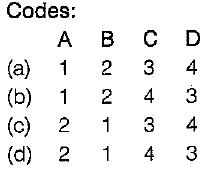Solution: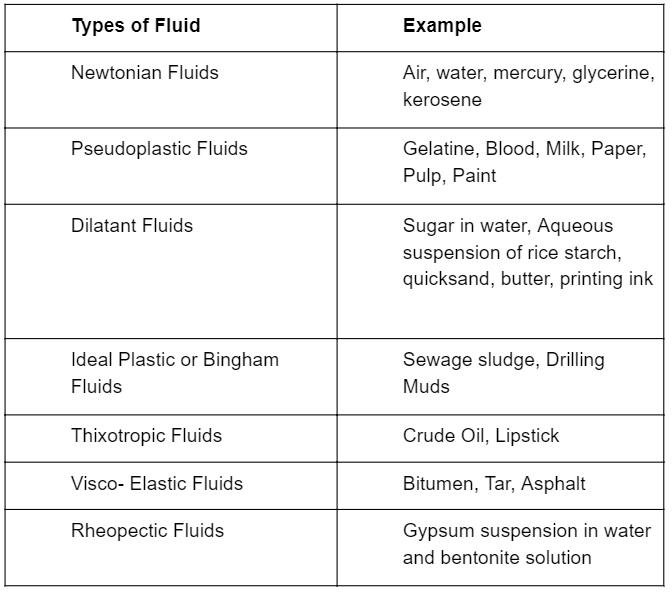QUESTION: 5

Match List-I (Definitions) with List-I I (Properties) and select the correct answer using the codes given below the lists:
List-I
A. Newtonian fluid
B. Ideal fluid
C. Thixotropic fluid
D. Rheological fluid

List-Il
1. Frictionless and incompressible
2. Viscosity is invariant with shear stress
3. Viscosity decreases at higher shear stress
4. Viscosity increases at higher shear stress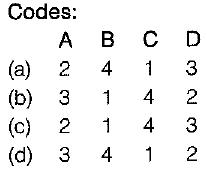Solution: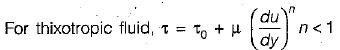As shear stress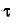increases viscosity increases with higher rates of shear strain.

QUESTION: 6

Mixing of milk with water is due to

Solution:

Adhesion- Two different particles binded together-Mixing of milk with water
Cohesion- Two same particles binded together-Mixing of water with water.

QUESTION: 7

Which one of the following expresses the height of rise or fall of a liquid in a capillary tube?

Solution:

Let us suppose that the level of liquid has risen (or fallen) by 'h' above (or below) the general liquid surface when a tube of diameter 'd' is inserted in the liquid. For equilibrium of vertical forces acting on the mass of liquid lying above (or below) the general liquid level, the weight of liquid column ‘h’ (or the total internal pressure in the case of capillary depression) must be balanced by the force, at surface of the liquid, due to surface tension σ. Thus, equating these two forces we have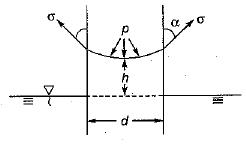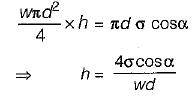QUESTION: 8

An ideal fluid is one which is

Solution:

Ideal fluid are those which have no viscosity and surface tension and are incompressible.

QUESTION: 9

Kinematic viscosity of gases on increase of temperature

Solution:

In case of gases the cohesion forces are small and molecular momentum transfer predominates. With the increase in temperature, molecular momentum transfer increases and hence viscosity increases.

QUESTION: 10

The liquid used in manometers should have

Solution:

The liquid used in manometer should be of light density so as to measure even small variation in pressure.